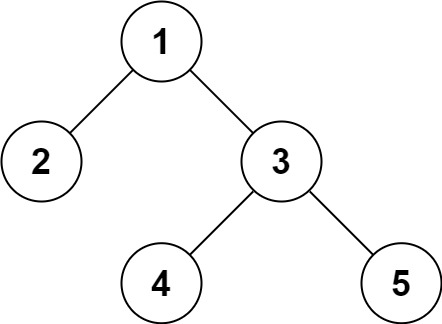# GeetCode Hub

Serialization is the process of converting a data structure or object into a sequence of bits so that it can be stored in a file or memory buffer, or transmitted across a network connection link to be reconstructed later in the same or another computer environment.

Design an algorithm to serialize and deserialize a binary tree. There is no restriction on how your serialization/deserialization algorithm should work. You just need to ensure that a binary tree can be serialized to a string and this string can be deserialized to the original tree structure.

Clarification: The input/output format is the same as how LeetCode serializes a binary tree. You do not necessarily need to follow this format, so please be creative and come up with different approaches yourself.

Example 1:```Input: root = [1,2,3,null,null,4,5]
Output: [1,2,3,null,null,4,5]
```

Example 2:

```Input: root = []
Output: []
```

Example 3:

```Input: root = 
Output: 
```

Example 4:

```Input: root = [1,2]
Output: [1,2]
```

Constraints:

• The number of nodes in the tree is in the range `[0, 104]`.
• `-1000 <= Node.val <= 1000`

/** * Definition for a binary tree node. * public class TreeNode { * int val; * TreeNode left; * TreeNode right; * TreeNode(int x) { val = x; } * } */ public class Codec { // Encodes a tree to a single string. public String serialize(TreeNode root) { } // Decodes your encoded data to tree. public TreeNode deserialize(String data) { } } // Your Codec object will be instantiated and called as such: // Codec ser = new Codec(); // Codec deser = new Codec(); // TreeNode ans = deser.deserialize(ser.serialize(root));